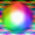## Friday, May 30, 2008

### Elearn Geometry Problem 112Area of Square and Triangle. Level: High School, SAT Prep, College geometry

1.You need additional points to prove this.

G - the center of semicircle
Then AG = DG = EG = FG = ½√S
And FG is perpendicular to CF
So knowing that CG = ½√(5S) lets us compute CF = √S = AB = AD = BC = CD

Using analytic coordinates, we can use the equations for CF = √S and FG = ½√S to prove that F is 1/5 of the way from A to D and 2/5 the way from A to B. Alternately, we can add the point H which is the closest point on AD to F, and use the similar 1:2:√5 triangles to prove the same idea.

Thus DE = √(S/2), DF = √(4S/5), and EF = √(S/10) which gives us the area of S/10

2.See

http://homepage2.nifty.com/tangoh/tan080616.html

Nice problem!!

3.If we take the midpoint of AC as the origin and label A:(-a,0),B:(-a,2a),C:(a,2a)& D:(a,0) then E is clearly (0,a). Now CF & CD are both tangents to the semicircle so CF = CD = 2a which means F is one of the intersections of the two circles given by x^2+y^2=a^2 &(x-a)^2+(y-2a)^2= 4a^2. This gives F as (-3a/5,4a/5).
Therefore, Tr. DEF =S1=(1/2)[a(a-4a/5)-(3a/5(-a)]
= 4a^2/10
But S = 2a*2a = 4a^2; hence S1 = S/10
Ajit: ajitathle@gmail.com

4.I tried a solution without coordinates in problem 112.
As triangles DEF and BEF have equal bases BE and DE and same altitude relative to that bases, then both have area S1. Being O the center of the semicircle, and s the side of the square ABCD, let’s write the altitude of triangle BAF as FG = p, the altitude of triangle AFD as FH = q, and ang(ADF) = a.
DOF is isosceles then ang(DOF) = 180º - 2a. Quadrilateral CDOF is cyclic, so ang(DCF) = 2a. We have CD = CF (tangents from C to the circle) thus CDOF is a kite, OC bisects the angle DCF and ang(DCO) = a.
We can see now that FHD and ODC are similar, with q/(s-p) = (s/2)/s so p = s - 2q (1).
Also AHF and AFD are similar, because ang(AFD) = 90º, so AHF and ODC are similar, with p/q = (s/2)/s and q = 2p (2).
From (1) and (2) we get p = s/5 and q = 2s/5.
Let S2 and S3 be the areas of AFD and AFB respectively. We have, successively
2S1 + S2 + S3 = S/2, then 2S1 + sq/2 + sp/2 = S/2, then 2S1 + (s^2)/5 + (s^2)/10 = S/2, then 2S1 + (3S)/10 = S/2, then S1 = S/10.

5.Let FD, OC meet at X

Then AFD, FXC,DXC are all congruent triangles and since each are similar to Tr.ODC, OC = sqrt5 a/ 2 where a = side of the square

Hence XD = a^2/4 /((sqrt5 a/2)/2) = a/sqrt5 = AF

S(AFD) = S(ODC) AF^2/OD ^2 = a^2/5

S(FCD) = 2a^2/5

So d the altitude from F in Tr. AFD = a^/5/(a/2) = 2a/5

So S(BFC) = (1/2)(3a/5)a = 3a^2/10

Hence S(BFD) = 2a^2/5+3a^2/10-a^2/2 = a^2/5

Therefore S(FED) = S(BFD)/2 = a^2/10 = S/10

Sumith Peiris
Moratuwa
Sri Lanka

6.Let the length of the square be 2 units
Join BF and AF and let m(CFE) = x
Hence m(FDE) = x (external segment theorem)

Since E is the center of the square, m(EAD) = m(EFD) = 45 -------(1)
Upon further angle chasing, we can deduce that C is the circumcenter of the triangle BFD
Hence m(BFE) = 135 and from (1) , m(BFE) = 90
In the right triangle BFE, we have
BF^2+FE^2 = BE^2
=> BF^2+FE^2=2 ---------(2)

Observe that the triangles, AFB and BFD are similar (AAA)
=> AB.FD=BD.BF
=> BF = FD/Sqrt(2) -------(3)

Now extend FE to a point P such that FP = 2.FE. Join DP and form the right isosceles triangle DPF (since BF_|_FE, by symmetry DP_|_FP and DP = BF)

Hence FE = FD/2.Sqrt(2) -----------(4)
Substitute (3) and (4) in (2)
=> FD^2=16/5 ---------(5)

Area of triangle FED = 0.5*(FE)*(DP)
=> S1 = 0.5*(FE)*(BF)
-> S1 = 0.5*(FD/2.Sqrt(2))*(FD/Sqrt(2)) [Substitute value of FD^2 from (5)]
=> S1 = 2/5 = 4/10 = 1/10(Area of the square ABCD)
Hence S1 = S/10

7.2nd Solution, much shorter.

Let S(AFD) = S2 & S(AFB) = S3

Since E is the mid point of BD, S(BFE) = S1.

Tr.s BFE & AFD are similar, so S2 = 2S1
Tr.s ABF & BFD are similar so S3/2S1 = 1/2 so S3 = S1

2S1 + S2 + S3 = S/2
So 2S1 + 2S1 + S1 = S/2 and hence
S1 = S/10

Sumith Peiris
Moratuwa
Sri Lanka